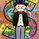43919 visningar
LazyBear's wavetrend oscillator enhanced with wavetrend cross visualization on the indicator as well as with bar color highlights.
```//
// @author LazyBear
//
// If you use this code in its original/modified form, do drop me a note.
//
study(title="WaveTrend with Crosses [LazyBear]", shorttitle="WT_CROSS_LB")
n1 = input(10, "Channel Length")
n2 = input(21, "Average Length")
obLevel1 = input(60, "Over Bought Level 1")
obLevel2 = input(53, "Over Bought Level 2")
osLevel1 = input(-60, "Over Sold Level 1")
osLevel2 = input(-53, "Over Sold Level 2")

ap = hlc3
esa = ema(ap, n1)
d = ema(abs(ap - esa), n1)
ci = (ap - esa) / (0.015 * d)
tci = ema(ci, n2)

wt1 = tci
wt2 = sma(wt1,4)

plot(0, color=gray)
plot(obLevel1, color=red)
plot(osLevel1, color=green)
plot(obLevel2, color=red)
plot(osLevel2, color=green)

plot(wt1, color=green)
plot(wt2, color=red)
plot(wt1-wt2, color=blue, style=area, transp=80)
plot(cross(wt1, wt2) ? wt2 : na, color = black , style = circles, linewidth = 3)
plot(cross(wt1, wt2) ? wt2 : na, color = (wt2 - wt1 > 0 ? red : lime) , style = circles, linewidth = 2)
barcolor(cross(wt1, wt2) ? (wt2 - wt1 > 0 ? aqua : yellow) : na)
```

## KommentarerCouple small changes. Removed the default colouring and titled each section for easier alerts. Also added an option to set an alert when the wavetrend is reversing.

n1 = input(10, "Channel Length")
n2 = input(21, "Average Length")
obLevel1 = input(60, "Over Bought Level 1")
obLevel2 = input(53, "Over Bought Level 2")
osLevel1 = input(-60, "Over Sold Level 1")
osLevel2 = input(-53, "Over Sold Level 2")

ap = hlc3
esa = ema(ap, n1)
d = ema(abs(ap - esa), n1)
ci = (ap - esa) / (0.015 * d)
tci = ema(ci, n2)

wt1 = tci
wt2 = sma(wt1,4)

plot(0, title="Zero Line")
plot(obLevel1, title="Overbought Lv.1")
plot(obLevel2, title="Overbought Lv.2")
plot(osLevel1, title="Oversold Lv.1")
plot(osLevel2, title="Oversold Lv.2")

plot(wt1, title="WT Bull Line")
plot(wt2, title="WT Bear Line")
plot(wt1-wt2, style=area, transp=80, title="Histo")
plot(cross(wt1, wt2) ? wt2 : na, color = black , style = circles, linewidth = 3, title="WT Crossing Outline")
plot(cross(wt1, wt2) ? wt2 : na, color = (wt2 - wt1 > 0 ? red : lime) , style = circles, linewidth = 2, title="WT Crossing Dots")

// Set Alerts for Bull/Bear Cross
wtCross = cross(wt1, wt2)
alertcondition(wtCross, title="Wave Trend Crossing", message="Wave Trend Crossing")
Svaramonnicore
@monnicore, amazing job, do you think it can work well on a daily and also 5 minutes chart? Best
SvaraJayosilia
@Jayosilia, Thanks. It all depends on how you use it, I don't think I would let it be my true entry/exit signal... rather, it adds confluence to my system.
Svararoycheng2016
@roycheng2016, Hey Roy, just draw a horizontal line on the indicator. Then in the alert dialog, you can set it to alert you when it crosses up/down the asset you're tracking.
Svara